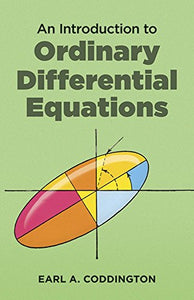An Introduction To Ordinary Differential Equations (Dover Books On Mathematics)

• Publish Date: 1989-03-01
• Binding: Paperback
• Author: Earl A. Coddington Mathematics
Regular price \$29.97 Sale price \$16.38

Attention: For textbook, access codes and supplements are not guaranteed with used items.

"Written in an admirably cleancut and economical style." Mathematical Reviews.
This concise text offers undergraduates in mathematics and science a thorough and systematic first course in elementary differential equations. Presuming a knowledge of basic calculus, the book first reviews the mathematical essentials required to master the materials to be presented.
The next four chapters take up linear equations, those of the first order and those with constant coefficients, variable coefficients, and regular singular points. The last two chapters address the existence and uniqueness of solutions to both first order equations and to systems and n-th order equations.
Throughout the book, the author carries the theory far enough to include the statements and proofs of the simpler existence and uniqueness theorems. Dr. Coddington, who has taught at MIT, Princeton, and UCLA, has included many exercises designed to develop the student's technique in solving equations. He has also included problems (with answers) selected to sharpen understanding of the mathematical structure of the subject, and to introduce a variety of relevant topics not covered in the text, e.g. stability, equations with periodic coefficients, and boundary value problems.